# Annotations#

Annotations are graphical elements, often pieces of text, that explain, add context to, or otherwise highlight some portion of the visualized data. `annotate` supports a number of coordinate systems for flexibly positioning data and annotations relative to each other and a variety of options of for styling the text. Axes.annotate also provides an optional arrow from the text to the data and this arrow can be styled in various ways. `text` can also be used for simple text annotation, but does not provide as much flexibility in positioning and styling as `annotate`.

## Basic annotation#

In an annotation, there are two points to consider: the location of the data being annotated xy and the location of the annotation text xytext. Both of these arguments are `(x, y)` tuples:

```import numpy as np
import matplotlib.pyplot as plt

fig, ax = plt.subplots(figsize=(3, 3))

t = np.arange(0.0, 5.0, 0.01)
s = np.cos(2*np.pi*t)
line, = ax.plot(t, s, lw=2)

ax.annotate('local max', xy=(2, 1), xytext=(3, 1.5),
arrowprops=dict(facecolor='black', shrink=0.05))
ax.set_ylim(-2, 2)
```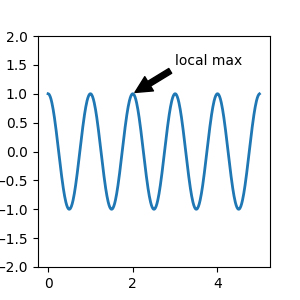In this example, both the xy (arrow tip) and xytext locations (text location) are in data coordinates. There are a variety of other coordinate systems one can choose -- you can specify the coordinate system of xy and xytext with one of the following strings for xycoords and textcoords (default is 'data')

argument

coordinate system

'figure points'

points from the lower left corner of the figure

'figure pixels'

pixels from the lower left corner of the figure

'figure fraction'

(0, 0) is lower left of figure and (1, 1) is upper right

'axes points'

points from lower left corner of axes

'axes pixels'

pixels from lower left corner of axes

'axes fraction'

(0, 0) is lower left of axes and (1, 1) is upper right

'data'

use the axes data coordinate system

The following strings are also valid arguments for textcoords

argument

coordinate system

'offset points'

offset (in points) from the xy value

'offset pixels'

offset (in pixels) from the xy value

For physical coordinate systems (points or pixels) the origin is the bottom-left of the figure or axes. Points are typographic points meaning that they are a physical unit measuring 1/72 of an inch. Points and pixels are discussed in further detail in Plotting in physical coordinates.

### Annotating data#

This example places the text coordinates in fractional axes coordinates:

```fig, ax = plt.subplots(figsize=(3, 3))

t = np.arange(0.0, 5.0, 0.01)
s = np.cos(2*np.pi*t)
line, = ax.plot(t, s, lw=2)

ax.annotate('local max', xy=(2, 1), xycoords='data',
xytext=(0.01, .99), textcoords='axes fraction',
va='top', ha='left',
arrowprops=dict(facecolor='black', shrink=0.05))
ax.set_ylim(-2, 2)
```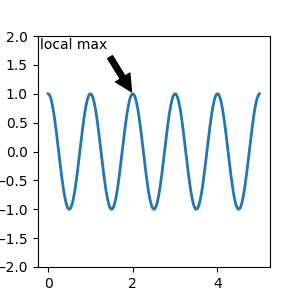### Annotating with arrows#

You can enable drawing of an arrow from the text to the annotated point by giving a dictionary of arrow properties in the optional keyword argument arrowprops.

arrowprops key

description

width

the width of the arrow in points

frac

the fraction of the arrow length occupied by the head

the width of the base of the arrow head in points

shrink

move the tip and base some percent away from the annotated point and text

**kwargs

any key for `matplotlib.patches.Polygon`, e.g., `facecolor`

In the example below, the xy point is in the data coordinate system since xycoords defaults to 'data'. For a polar axes, this is in (theta, radius) space. The text in this example is placed in the fractional figure coordinate system. `matplotlib.text.Text` keyword arguments like horizontalalignment, verticalalignment and fontsize are passed from `annotate` to the `Text` instance.

```fig = plt.figure()
r = np.arange(0, 1, 0.001)
theta = 2 * 2*np.pi * r
line, = ax.plot(theta, r, color='#ee8d18', lw=3)

ind = 800
thisr, thistheta = r[ind], theta[ind]
ax.plot([thistheta], [thisr], 'o')
ax.annotate('a polar annotation',
xytext=(0.05, 0.05),    # fraction, fraction
textcoords='figure fraction',
arrowprops=dict(facecolor='black', shrink=0.05),
horizontalalignment='left',
verticalalignment='bottom')
```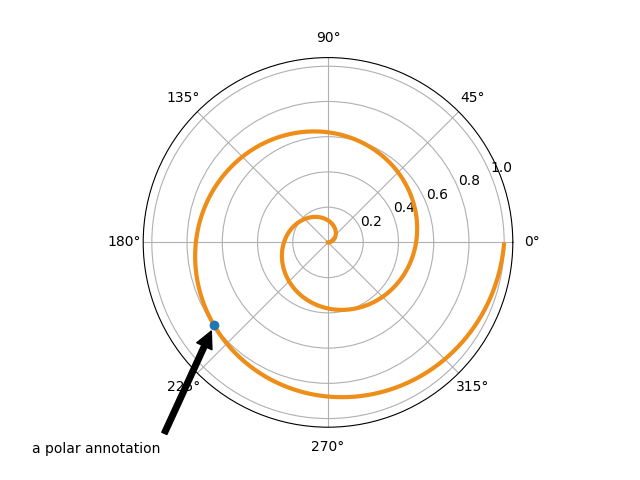For more on plotting with arrows, see Customizing annotation arrows

### Placing text annotations relative to data#

Annotations can be positioned at a relative offset to the xy input to annotation by setting the textcoords keyword argument to `'offset points'` or `'offset pixels'`.

```fig, ax = plt.subplots(figsize=(3, 3))
x = [1, 3, 5, 7, 9]
y = [2, 4, 6, 8, 10]
annotations = ["A", "B", "C", "D", "E"]
ax.scatter(x, y, s=20)

for xi, yi, text in zip(x, y, annotations):
ax.annotate(text,
xy=(xi, yi), xycoords='data',
xytext=(1.5, 1.5), textcoords='offset points')
```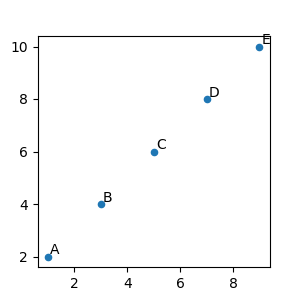The annotations are offset 1.5 points (1.5*1/72 inches) from the xy values.

We recommend reading Basic annotation, `text()` and `annotate()` before reading this section.

### Annotating with boxed text#

`text` takes a bbox keyword argument, which draws a box around the text:

```fig, ax = plt.subplots(figsize=(5, 5))
t = ax.text(0.5, 0.5, "Direction",
ha="center", va="center", rotation=45, size=15,
fc="lightblue", ec="steelblue", lw=2))
```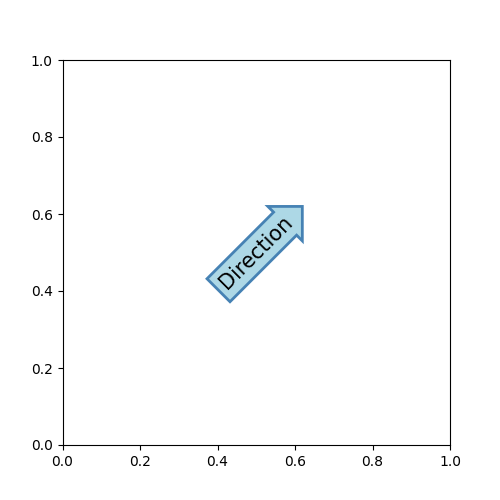The arguments are the name of the box style with its attributes as keyword arguments. Currently, following box styles are implemented.

Class

Name

Attrs

Circle

`circle`

DArrow

`darrow`

Ellipse

`ellipse`

LArrow

`larrow`

RArrow

`rarrow`

Round

`round`

Round4

`round4`

Roundtooth

`roundtooth`

Sawtooth

`sawtooth`

Square

`square`

The patch object (box) associated with the text can be accessed using:

```bb = t.get_bbox_patch()
```

The return value is a `FancyBboxPatch`; patch properties (facecolor, edgewidth, etc.) can be accessed and modified as usual. `FancyBboxPatch.set_boxstyle` sets the box shape:

```bb.set_boxstyle("rarrow", pad=0.6)
```

The attribute arguments can also be specified within the style name with separating comma:

```bb.set_boxstyle("rarrow, pad=0.6")
```

### Defining custom box styles#

You can use a custom box style. The value for the `boxstyle` can be a callable object in the following forms:

```from matplotlib.path import Path

def custom_box_style(x0, y0, width, height, mutation_size):
"""
Given the location and size of the box, return the path of the box around
it. Rotation is automatically taken care of.

Parameters
----------
x0, y0, width, height : float
Box location and size.
mutation_size : float
Mutation reference scale, typically the text font size.
"""
width = width + 2 * pad
height = height + 2 * pad
# boundary of the padded box
x1, y1 = x0 + width, y0 + height
# return the new path
return Path([(x0, y0), (x1, y0), (x1, y1), (x0, y1),
(x0-pad, (y0+y1)/2), (x0, y0), (x0, y0)],
closed=True)

fig, ax = plt.subplots(figsize=(3, 3))
ax.text(0.5, 0.5, "Test", size=30, va="center", ha="center", rotation=30,
bbox=dict(boxstyle=custom_box_style, alpha=0.2))
```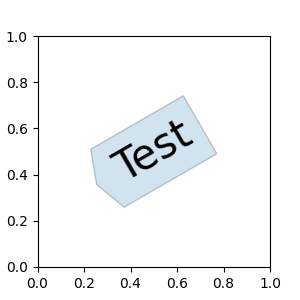See also Custom box styles. Similarly, you can define a custom `ConnectionStyle` and a custom `ArrowStyle`. View the source code at `patches` to learn how each class is defined.

### Customizing annotation arrows#

An arrow connecting xy to xytext can be optionally drawn by specifying the arrowprops argument. To draw only an arrow, use empty string as the first argument:

```fig, ax = plt.subplots(figsize=(3, 3))
ax.annotate("",
xy=(0.2, 0.2), xycoords='data',
xytext=(0.8, 0.8), textcoords='data',
arrowprops=dict(arrowstyle="->", connectionstyle="arc3"))
```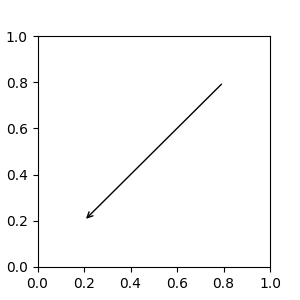The arrow is drawn as follows:

1. A path connecting the two points is created, as specified by the connectionstyle parameter.

2. The path is clipped to avoid patches patchA and patchB, if these are set.

3. The path is further shrunk by shrinkA and shrinkB (in pixels).

4. The path is transmuted to an arrow patch, as specified by the arrowstyle parameter.

The creation of the connecting path between two points is controlled by `connectionstyle` key and the following styles are available.

Name

Attrs

`angle`

`angle3`

angleA=90,angleB=0

`arc`

`arc3`

`bar`

armA=0.0,armB=0.0,fraction=0.3,angle=None

Note that "3" in `angle3` and `arc3` is meant to indicate that the resulting path is a quadratic spline segment (three control points). As will be discussed below, some arrow style options can only be used when the connecting path is a quadratic spline.

The behavior of each connection style is (limitedly) demonstrated in the example below. (Warning: The behavior of the `bar` style is currently not well-defined and may be changed in the future).

The connecting path (after clipping and shrinking) is then mutated to an arrow patch, according to the given `arrowstyle`.

Name

Attrs

`-`

None

`->`

`-[`

widthB=1.0,lengthB=0.2,angleB=None

`|-|`

widthA=1.0,widthB=1.0

`-|>`

`<-`

`<->`

`<|-`

`<|-|>`

`fancy`

`simple`

`wedge`

tail_width=0.3,shrink_factor=0.5

Some arrowstyles only work with connection styles that generate a quadratic-spline segment. They are `fancy`, `simple`, and `wedge`. For these arrow styles, you must use the "angle3" or "arc3" connection style.

If the annotation string is given, the patch is set to the bbox patch of the text by default.

```fig, ax = plt.subplots(figsize=(3, 3))

ax.annotate("Test",
xy=(0.2, 0.2), xycoords='data',
xytext=(0.8, 0.8), textcoords='data',
size=20, va="center", ha="center",
arrowprops=dict(arrowstyle="simple",
```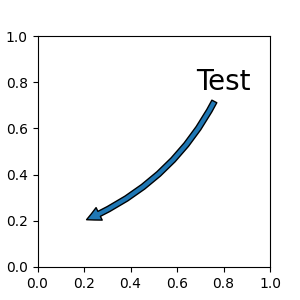As with `text`, a box around the text can be drawn using the bbox argument.

```fig, ax = plt.subplots(figsize=(3, 3))

ann = ax.annotate("Test",
xy=(0.2, 0.2), xycoords='data',
xytext=(0.8, 0.8), textcoords='data',
size=20, va="center", ha="center",
bbox=dict(boxstyle="round4", fc="w"),
arrowprops=dict(arrowstyle="-|>",
fc="w"))
```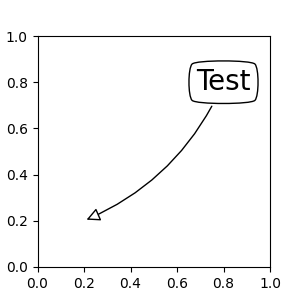By default, the starting point is set to the center of the text extent. This can be adjusted with `relpos` key value. The values are normalized to the extent of the text. For example, (0, 0) means lower-left corner and (1, 1) means top-right.

```fig, ax = plt.subplots(figsize=(3, 3))

ann = ax.annotate("Test",
xy=(0.2, 0.2), xycoords='data',
xytext=(0.8, 0.8), textcoords='data',
size=20, va="center", ha="center",
bbox=dict(boxstyle="round4", fc="w"),
arrowprops=dict(arrowstyle="-|>",
relpos=(0., 0.),
fc="w"))

ann = ax.annotate("Test",
xy=(0.2, 0.2), xycoords='data',
xytext=(0.8, 0.8), textcoords='data',
size=20, va="center", ha="center",
bbox=dict(boxstyle="round4", fc="w"),
arrowprops=dict(arrowstyle="-|>",
relpos=(1., 0.),
fc="w"))
```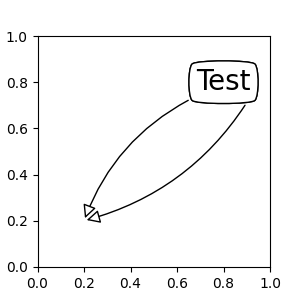### Placing Artist at anchored Axes locations#

There are classes of artists that can be placed at an anchored location in the Axes. A common example is the legend. This type of artist can be created by using the `OffsetBox` class. A few predefined classes are available in `matplotlib.offsetbox` and in `mpl_toolkits.axes_grid1.anchored_artists`.

```from matplotlib.offsetbox import AnchoredText

fig, ax = plt.subplots(figsize=(3, 3))
at = AnchoredText("Figure 1a",
prop=dict(size=15), frameon=True, loc='upper left')
```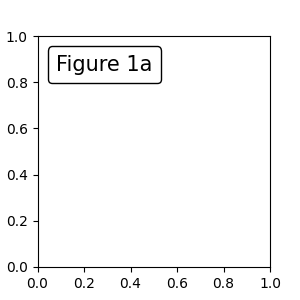The loc keyword has same meaning as in the legend command.

A simple application is when the size of the artist (or collection of artists) is known in pixel size during the time of creation. For example, If you want to draw a circle with fixed size of 20 pixel x 20 pixel (radius = 10 pixel), you can utilize `AnchoredDrawingArea`. The instance is created with a size of the drawing area (in pixels), and arbitrary artists can be added to the drawing area. Note that the extents of the artists that are added to the drawing area are not related to the placement of the drawing area itself. Only the initial size matters.

The artists that are added to the drawing area should not have a transform set (it will be overridden) and the dimensions of those artists are interpreted as a pixel coordinate, i.e., the radius of the circles in above example are 10 pixels and 5 pixels, respectively.

```from matplotlib.patches import Circle
from mpl_toolkits.axes_grid1.anchored_artists import AnchoredDrawingArea

fig, ax = plt.subplots(figsize=(3, 3))
ada = AnchoredDrawingArea(40, 20, 0, 0,
p1 = Circle((10, 10), 10)
p2 = Circle((30, 10), 5, fc="r")
```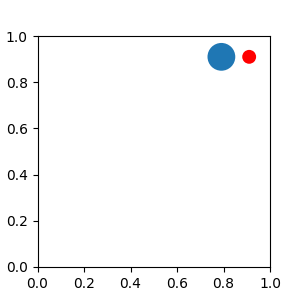Sometimes, you want your artists to scale with the data coordinate (or coordinates other than canvas pixels). You can use `AnchoredAuxTransformBox` class. This is similar to `AnchoredDrawingArea` except that the extent of the artist is determined during the drawing time respecting the specified transform.

The ellipse in the example below will have width and height corresponding to 0.1 and 0.4 in data coordinates and will be automatically scaled when the view limits of the axes change.

```from matplotlib.patches import Ellipse
from mpl_toolkits.axes_grid1.anchored_artists import AnchoredAuxTransformBox

fig, ax = plt.subplots(figsize=(3, 3))
box = AnchoredAuxTransformBox(ax.transData, loc='upper left')
el = Ellipse((0, 0), width=0.1, height=0.4, angle=30)  # in data coordinates!
```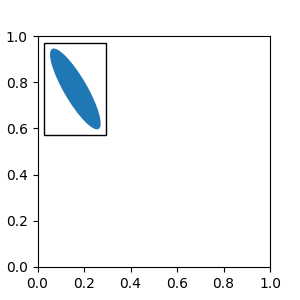Another method of anchoring an artist relative to a parent axes or anchor point is via the bbox_to_anchor argument of `AnchoredOffsetbox`. This artist can then be automatically positioned relative to another artist using `HPacker` and `VPacker`:

```from matplotlib.offsetbox import (AnchoredOffsetbox, DrawingArea, HPacker,
TextArea)

fig, ax = plt.subplots(figsize=(3, 3))

box1 = TextArea(" Test: ", textprops=dict(color="k"))
box2 = DrawingArea(60, 20, 0, 0)

el1 = Ellipse((10, 10), width=16, height=5, angle=30, fc="r")
el2 = Ellipse((30, 10), width=16, height=5, angle=170, fc="g")
el3 = Ellipse((50, 10), width=16, height=5, angle=230, fc="b")

box = HPacker(children=[box1, box2],
align="center",

anchored_box = AnchoredOffsetbox(loc='lower left',
frameon=True,
bbox_to_anchor=(0., 1.02),
bbox_transform=ax.transAxes,

```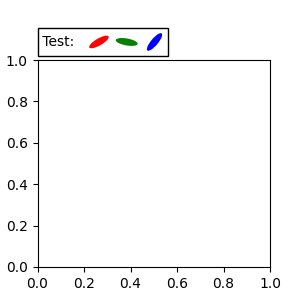Note that, unlike in `Legend`, the `bbox_transform` is set to `IdentityTransform` by default

## Coordinate systems for annotations#

Matplotlib Annotations support several types of coordinate systems. The examples in Basic annotation used the `data` coordinate system; Some others more advanced options are:

1. A `Transform` instance. For more information on transforms, see the Transformations Tutorial For example, the `Axes.transAxes` transform positions the annotation relative to the Axes coordinates and using it is therefore identical to setting the coordinate system to "axes fraction":

```fig, (ax1, ax2) = plt.subplots(nrows=1, ncols=2, figsize=(6, 3))
ax1.annotate("Test", xy=(0.5, 0.5), xycoords=ax1.transAxes)
ax2.annotate("Test", xy=(0.5, 0.5), xycoords="axes fraction")
```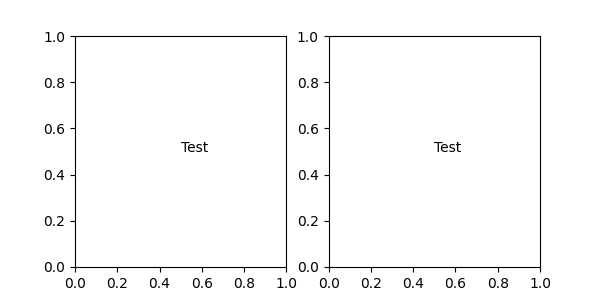Another commonly used `Transform` instance is `Axes.transData`. This transform is the coordinate system of the data plotted in the axes. In this example, it is used to draw an arrow from a point in ax1 to text in ax2, where the point and text are positioned relative to the coordinates of ax1 and ax2 respectively:

```fig, (ax1, ax2) = plt.subplots(nrows=1, ncols=2, figsize=(6, 3))

ax1.annotate("Test1", xy=(0.5, 0.5), xycoords="axes fraction")
ax2.annotate("Test2",
xy=(0.5, 0.5), xycoords=ax1.transData,
xytext=(0.5, 0.5), textcoords=ax2.transData,
arrowprops=dict(arrowstyle="->"))
```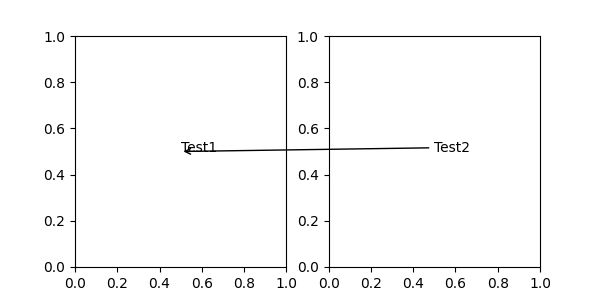2. An `Artist` instance. The xy value (or xytext) is interpreted as a fractional coordinate of the bounding box (bbox) of the artist:

```fig, ax = plt.subplots(nrows=1, ncols=1, figsize=(3, 3))
an1 = ax.annotate("Test 1",
xy=(0.5, 0.5), xycoords="data",
va="center", ha="center",
bbox=dict(boxstyle="round", fc="w"))

an2 = ax.annotate("Test 2",
xy=(1, 0.5), xycoords=an1,  # (1, 0.5) of an1's bbox
xytext=(30, 0), textcoords="offset points",
va="center", ha="left",
bbox=dict(boxstyle="round", fc="w"),
arrowprops=dict(arrowstyle="->"))
```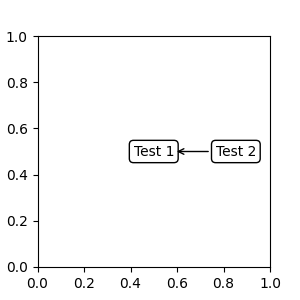Note that you must ensure that the extent of the coordinate artist (an1 in this example) is determined before an2 gets drawn. Usually, this means that an2 needs to be drawn after an1. The base class for all bounding boxes is `BboxBase`

3. A callable object that takes the renderer instance as single argument, and returns either a `Transform` or a `BboxBase`. For example, the return value of `Artist.get_window_extent` is a bbox, so this method is identical to (2) passing in the artist:

```fig, ax = plt.subplots(nrows=1, ncols=1, figsize=(3, 3))
an1 = ax.annotate("Test 1",
xy=(0.5, 0.5), xycoords="data",
va="center", ha="center",
bbox=dict(boxstyle="round", fc="w"))

an2 = ax.annotate("Test 2",
xy=(1, 0.5), xycoords=an1.get_window_extent,
xytext=(30, 0), textcoords="offset points",
va="center", ha="left",
bbox=dict(boxstyle="round", fc="w"),
arrowprops=dict(arrowstyle="->"))
````Artist.get_window_extent` is the bounding box of the Axes object and is therefore identical to setting the coordinate system to axes fraction:

```fig, (ax1, ax2) = plt.subplots(nrows=1, ncols=2, figsize=(6, 3))

an1 = ax1.annotate("Test1", xy=(0.5, 0.5), xycoords="axes fraction")
an2 = ax2.annotate("Test 2", xy=(0.5, 0.5), xycoords=ax2.get_window_extent)
```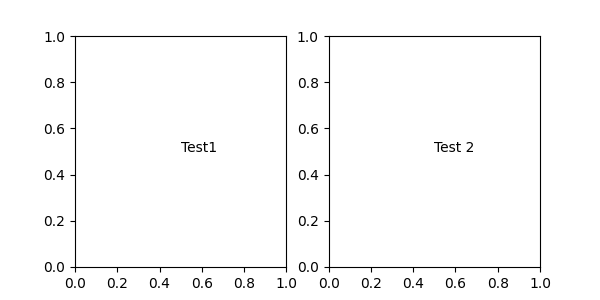4. A blended pair of coordinate specifications -- the first for the x-coordinate, and the second is for the y-coordinate. For example, x=0.5 is in data coordinates, and y=1 is in normalized axes coordinates:

```fig, ax = plt.subplots(figsize=(3, 3))
ax.annotate("Test", xy=(0.5, 1), xycoords=("data", "axes fraction"))
ax.axvline(x=.5, color='lightgray')
ax.set(xlim=(0, 2), ylim=(1, 2))
```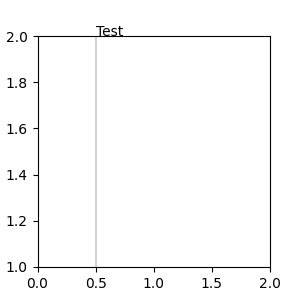5. Sometimes, you want your annotation with some "offset points", not from the annotated point but from some other point or artist. `text.OffsetFrom` is a helper for such cases.

```from matplotlib.text import OffsetFrom

fig, ax = plt.subplots(figsize=(3, 3))
an1 = ax.annotate("Test 1", xy=(0.5, 0.5), xycoords="data",
va="center", ha="center",
bbox=dict(boxstyle="round", fc="w"))

offset_from = OffsetFrom(an1, (0.5, 0))
an2 = ax.annotate("Test 2", xy=(0.1, 0.1), xycoords="data",
xytext=(0, -10), textcoords=offset_from,
# xytext is offset points from "xy=(0.5, 0), xycoords=an1"
va="top", ha="center",
bbox=dict(boxstyle="round", fc="w"),
arrowprops=dict(arrowstyle="->"))
```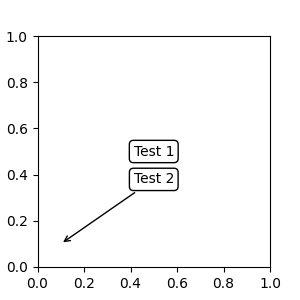### Using ConnectionPatch#

`ConnectionPatch` is like an annotation without text. While `annotate` is sufficient in most situations, `ConnectionPatch` is useful when you want to connect points in different axes. For example, here we connect the point xy in the data coordinates of `ax1` to point xy in the data coordinates of `ax2`:

```from matplotlib.patches import ConnectionPatch

fig, (ax1, ax2) = plt.subplots(nrows=1, ncols=2, figsize=(6, 3))
xy = (0.3, 0.2)
con = ConnectionPatch(xyA=xy, coordsA=ax1.transData,
xyB=xy, coordsB=ax2.transData)

```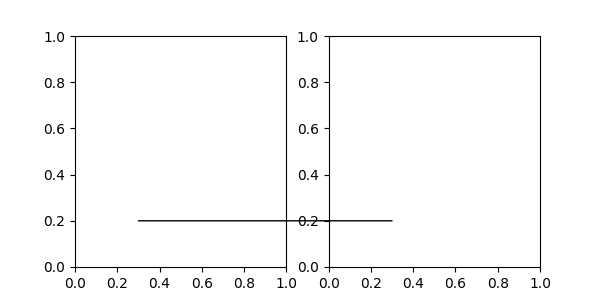Here, we added the `ConnectionPatch` to the figure (with `add_artist`) rather than to either axes. This ensures that the ConnectionPatch artist is drawn on top of both axes, and is also necessary when using constrained_layout for positioning the axes.

### Zoom effect between Axes#

`mpl_toolkits.axes_grid1.inset_locator` defines some patch classes useful for interconnecting two axes.

The code for this figure is at Axes Zoom Effect and familiarity with Transformations Tutorial is recommended.

Total running time of the script: ( 0 minutes 3.874 seconds)

Gallery generated by Sphinx-Gallery